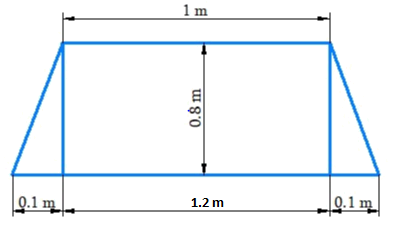# Ex.11.2 Q1 Mensuration Solution - NCERT Maths Class 8

## Question

The shape of the top surface of a table is a trapezium. Find its area if its parallel sides are $$1\,\rm{ m}$$ and $$1.2\,\rm{ m}$$ and perpendicular distance between them is $$0.8\rm{ m}.$$Video Solution
Mensuration
Ex 11.2 | Question 1

## Text Solution

What is Known?

Shape of the table is trapezium and dimensions of the table.

What is unknown?

Area of the table.

Reasoning:

The table is rectangular in the middle and triangle at the end. Usually the area of the problem is sum of areas of two right angle triangle and rectangle.

Steps:

Base of the triangles

\begin{align}(CE+FD) &= (CD-EF)\\&= 1.2\;\rm{cm} – 1\,\rm{m} \\&= 0.2\,\rm{m}\end{align}

Base of one triangle \begin{align} = {\rm{CE}} = {\rm{FD}} = \frac{{0.2}}{2} = 0.1{\rm{m}} \end{align}

Height of the triangle $$= AE = BF = 0.3\,\rm{m}$$

Area of the triangle $$ACE$$ $$=$$ Area of the triangle $$BDF$$
\begin{align} &= \frac{1}{2} \times \rm{base} \times \rm{height}\\& = \frac{1}{2} \times 0.1{\rm{m}} \times 0.8{\rm{m}} = 0.04{{\rm{m}}^2}\end{align}

Area of the rectangle $$ABEF$$
\begin{align} &= 1{\rm{m}} \times 0.8{\rm{m}}\\ &= 0.8\,\rm{m^2}\end{align}

Therefore,
Area of the table $$=$$ Area of the triangle $$ACE$$ $$+$$ Area of the rectangle $$ABEF$$ $$+$$ Area of the triangle $$BFD$$

\begin{align}&= 0.04\,{{\rm{m}}^2} \times 0.8\,{{\rm{m}}^2} + 0.04\,{{\rm{m}}^2}\\&= 0.88\,{{\rm{m}}^2}\end{align}

Learn from the best math teachers and top your exams

• Live one on one classroom and doubt clearing
• Practice worksheets in and after class for conceptual clarity
• Personalized curriculum to keep up with school